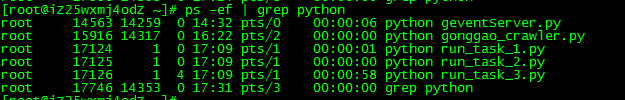### Python程序的几种退出方式

Python 核心编程（第二版）

http://www.zhihu.com/question/21187839

1. sys.exit()

```1 import sys
2 sys.exit()
3 sys.exit(0)
4 sys.exit(1)```

2. os._exit()

3. os.kill()

SIGINT      终止进程     中断进程

SIGTERM   终止进程     软件终止信号

SIGKILL    终止进程      杀死进程

SIGALRM   闹钟信号~~~~~~~~~~~~~~~~~~~~~~~~~~~~~~~~~~~~~~~~~~~~~~~~~~~~~~~~~~~~~~~~~~~~~~~~~~~~~~~~~

`#! /usr/bin/python`
`#　-*- coding: utf-8 -*-`

`import`
`os`
`import`
`sys`
`import`
`signal`

`def`
`kill(pid):`

`  `
`try`
`:`
`    `
`a `
`=`
`os.kill(pid, signal.SIGKILL)`
`    `
`# a = os.kill(pid, signal.9) #　与上等效`
`    `
`print`
`'已杀死pid为%s的进程,　返回值是:%s'`
`%`
`(pid, a)`
`  `
`except`
`OSError, e:`
`    `
`print`
`'没有如此进程!!!'`

`if`
`__name__ `
`=`
`=`
`'__main__'`
`:`
`  `
`kill(`
`8132`
`)`

~~~~~~~~~~~~~~~~~~~~~~~~[python]
view plain
copy

1. #coding=utf-8
2. import sys,os
3. def kill_crawler(id):
4.     cmd = ‘ps -ef | grep python’
5.     f = os.popen(cmd)
7.     for line in txt:
8.         colum = line.split()
9.         pid = colum
10.     name = colum[-1]
13.         if task_id == id or id ==‘0’:
14.         cmd = “kill -9 %d” % int(pid)
15.         rc = os.system(cmd)
16.         if rc == 0 :
17.                     print “stop \”%s\” success!!” % name
18.                 else:
19.                     print “stop \”%s\” failed!!” % name
20.
21. if __name__ ==‘__main__’:
22.
23.     if not  len(sys.argv)==2:
24.     print u‘输入要结束的任务编号，0代表停止所有’
25.         sys.exit()
26.     id = sys.argv
27.     kill_crawler(id)

~~~~~~~~~~~~~~~~~~~~~~~~~~~~~~~~~~~~~~~~~~~~~~~~~~~~~~~~~~~~~~

4. Windows下Kill进程

```1 import os